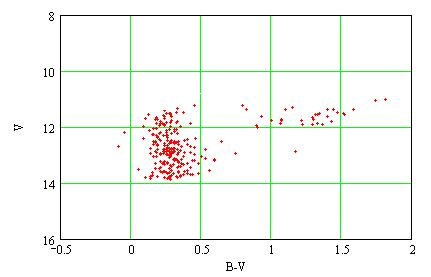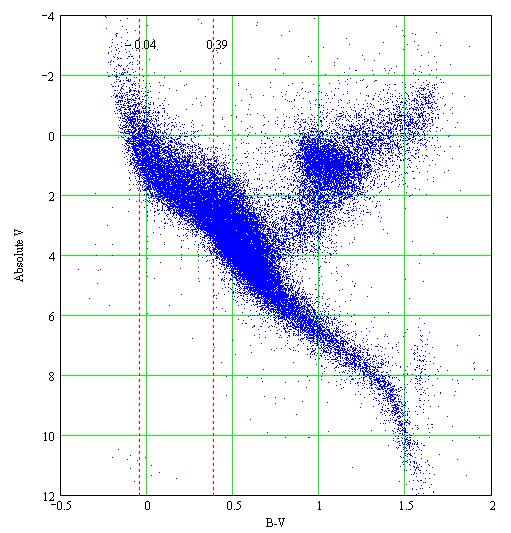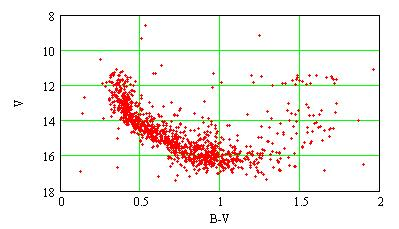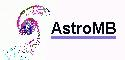MBCAA Observatory

# M11: open cluster distance measurement

## Observed: 23 June 2006

### Abstract

The M11 open cluster (Wild Duck cluster, NGC6705) is observed through B and V filters. An HR diagram is constructed. It is compared with an absolute HR diagram made from Hipparcos data which measured the parallaxes of nearby stars. The distance to M11 is then derived. The interstellar extinction is taken into account using the Henden's sequence for M11 (U data).

### Observations

I observed M11 with a 203mm f/6.3 SC telescope, a SBIG ST7E camera (KAF401E CCD) and Johnson B and V filters. There is one 200s exposure through each filter. The images are HERE.

The images are analyzed with the AstroMB software program that detects the stars and measures their intensities by fitting each of them with a PSF (a Gaussian). The star magnitudes are computed by comparing them with the Tycho catalog (the VT and BT magnitudes of the Tycho catalog are converted into the Johnson V and B magnitudes using Mamajek et al (2002)).

For the V filter image, 866 stars are detected with S/N>=5, 41 Tycho stars are used as comparison stars with an average dispersion around the Pogson's law of 0.26 mag (0.79 max).
For the B filter image, 324 stars are detected, 40 Tycho comparison stars are used, with an average dispersion of 0.20 mag (0.56 max).

The stars detected with the V and B filters are matched with a dedicated software program (UT_M11.exe). 280 stars are detected on both the V and B images within 7 arcminutes from the cluster center. An Hertzsprung-Russell diagram (V versus the color B-V) can be construsted:The average magnitudes are <V>=12.52 and <B-V>=0.39.

### Absolute HR diagram

An absolute Hertzsprung-Russell diagram is built from the data of the Hipparcos satellite (ESA (1997)). This satellite accuratly measured the parallaxes of nearby stars (then the distances) along with their magnitudes. 118218 stars were observed. I use only the stars with a precision on their parallax measurements better than 20%, ie 49239 stars. The resulting HR diagram for absolute V magnitudes (magnitudes for the stars at 10 pc) versus B-V colors is:The mark at 0.39 is my measure of <B-V>. The mark at -0.04 is <B-V> corrected for the interstellar extinction (see below).

### First estimation of the distance

My measure of <B-V> matches the absolute magnitude MV=2.64 on the Hipparcos HR diagram. The distance is then:

d = 10^(<V> - MV + 5)/5 = 1.0 kpc.

However, with a distance this large, the interstellar extinction should be taken into account:

### Interstellar extinction

The interstellar extinction is caracterized by the R parameter defined as:

R = AV/E(B-V)

with AV the dimming in V, and E(B-V) the reddening (in magnitudes). For the Milky Way R=3.1.

The color-color diagrams, U-B versus B-V, of star clusters show a minimum at B-V=0.17 and a maximum at B-V=0.47 (Mermilliod (1981)).

Then the interstellar extinction of M11 may be taken into account the following way:

• observe M11 with a U filter;
• make a color-color diagram, observe the minimum and the maximum. Let be x the minimum. The reddening is then E(B-V)=x-0.17;
• compute the dimming in V: AV=R*E(B-V);
• correct the <B-V> magnitudes for E(B-V);
• correct the <V> magnitudes for AV;
• match the corrected <B-V> and <V> magnitudes with the HR diagram in absolute MV and derive the distance.
• I was not able to observe M11 with a U filter. I use then the Henden's sequence for M11. In this sequence of 2069 stars, 1160 are measured in U, B, V. The resulting color-color diagram shows a minimum around B-V=0.6 and a maximum around B-V=0.9:Then: E(B-V) = 0.6-0.17 = 0.43
AV = R*E(B-V) = 3.1*0.43 = 1.33

The average magnitudes that I measured become with these corrections:

<V> = 12.52-1.33 = 11.19
<B-V> = 0.39-0.43 = -0.04

On the Hipparcos HR diagram (which has no extinction because it is derived from nearby stars) this value of <B-V> corresponds to MV=0.36 and the distance is:

d = 10^(<V>-MV+5)/5 = 1.5 kpc

### Discussion

After correction for the interstellar extinction, M11 appears as being quite blue (which implies it is very young). This part of the Hipparcos HR diagram is rather steep and the incertainty in B-V (from my measurement and from the color-color diagram minimum) translates into a large one in V.

From the Henden's sequence, that goes to fainter magnitudes than my observations, the following HR diagram is derived:with <B-V>=0.76 and <V>=14.68. This corresponds to MV=4.75 on the Hipparcos HR diagram, then to the distance d=1.0kpc.
With the interstellar extinction, one has <B-V>=0.76-0.43=0.33 and <V>=14.68-1.33=15. Then MV=2.31 and d=1.6kpc.

This kind of distance measurement was already done at professionnal observatories, of course. For example Sung et al (1999) used a theoretical model for stellar evolution (instead of an absolute HR diagram) to derive a distance of 2.0+/-0.1 kpc and an age of 250 Myr.

### Acknowledgement

The use of Henden's sequence for M11, available from ftp://ftp.aavso.org/public/calib/, is acknowledged.

### References

ESA (1997) The Hipparcos and Tycho catalogues SP-1200.

Mamajek E., Meyer M., Liebert J. (2002) AJ 124 1670M (appendix C).

Mermilliod J.C. (1981) A&A 97 235.

Sung H., Bessell M.S., Lee H., Kang Y.H., Lee S. (1999) MNRAS 310 982.

### Technical notes

Telescope and camera configuration.Software for astronomy
 Site map Search
 .